1.If $\mid z_{1}\mid =\mid z_{2}\mid=\mid z_{3}\mid=1$     and $z_{1}+z_{2}+z_{3}=\sqrt{2}+i$     , then the complex number $z_{2}\bar{z}_{3}+z_{3}\bar{z}_{1}+z_{1}\bar{z}_{2}$     is
a) purely imaginary
b) purely real
c) positive real number
d) none of these

Explanation: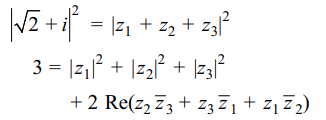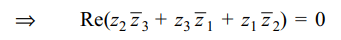2. If a, b, c are distinct integers and $\omega\neq 1$  is a cube root of unity then minimum value of $x=\mid a+b\omega+c\omega^{2}\mid+\mid a+b\omega^{2}+c\omega\mid$
a) $2\sqrt{3}$
b) 3
c) $4\sqrt{2}$
d) 2

Explanation: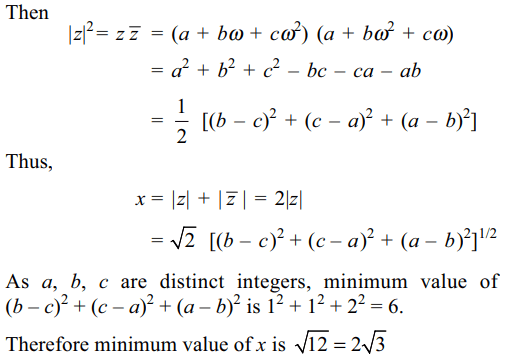3.Let f (x) be a polynomial with real coefficient such that $\left(x^{3}-a^{3}\right)\mid \left(f(x)-f\left(a\right)\right)$     for each positive real number a, then f (x) is of the form
a) $p\left(x^{3}\right)$
b) $xp\left(x^{3}\right)$
c) $x^{2}p\left(x^{3}\right)$
d) a constant

Explanation: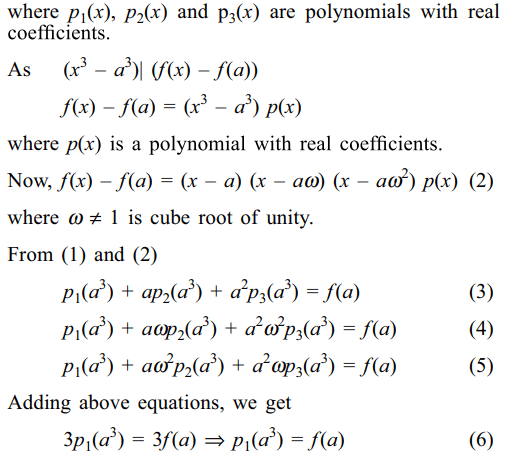4. Suppose m, n, $\epsilon$  R - {0} and $m \neq n$   . If incentre of triangle with vertices A(m, 0), B(0, n) and $C\left(\alpha ,-\alpha\right)$   is the origin, then $\alpha$ is equal to
a) $\frac{mn}{n-m}$
b) $\frac{mn}{m-n}$
c) 0
d) $\frac{mn}{\mid m-n\mid}$

Explanation: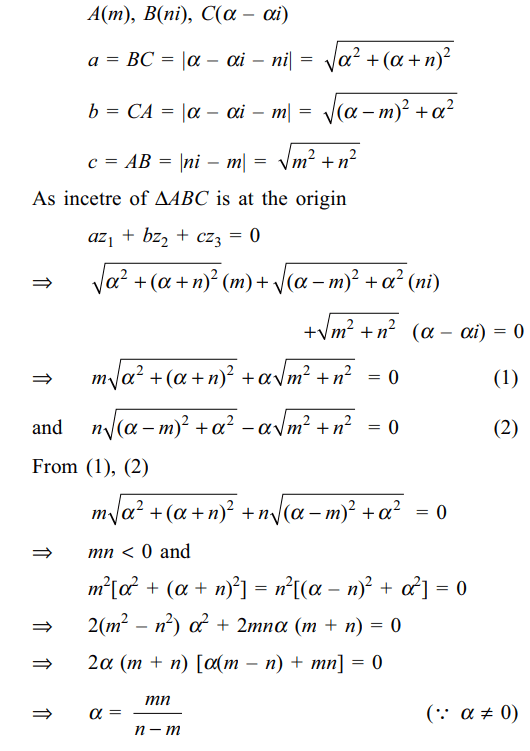5. Let $r_{k}>0$  and $z_{k}=r_{k}\left(\cos\alpha _{k}+i \sin\alpha _{k}\right)$     for k = 1, 2, 3 be such that
$\frac{1}{z_{1}}+\frac{1}{z_{2}}+\frac{1}{z_{3}}=0$
Let Ak be the point in the complex plane given by $w_{k}=\frac{\cos2\alpha _{k}+i \sin2\alpha _{k}}{z_{k}}$     for k = 1, 2, 3. The origin, O is the
a) incentre of $\triangle A_{1}A_{2}A_{3}$
b) orthocentre of $\triangle A_{1}A_{2}A_{3}$
c) circumcentre of $\triangle A_{1}A_{2}A_{3}$
d) centroid of $\triangle A_{1}A_{2}A_{3}$

Explanation: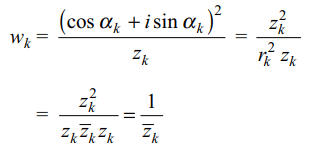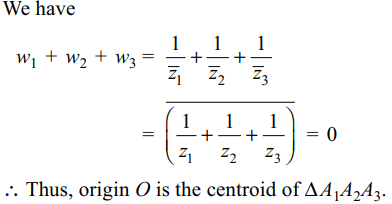6. If a and b are two real numbers and $z_{1}$ and $z_{2}$ are two non zero complex numbers such that $a \mid z_{1}\mid=b \mid z_{2}\mid$    then $z=\frac{az_{1}}{bz_{2}}+\frac{bz_{2}}{az_{1}}$
a) z is purely real
b) z is purely imaginary
c) $\mid z\mid=a/b$
d) none of these

Explanation: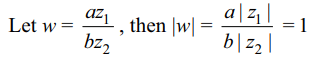7. $z_{1}$ and $z_{2}$ lie on a circle with centre at the origin. The point of intersection z3 of the tangents at $z_{1}$ and $z_{2}$ is given by
a) $\frac{1}{2}\left(\bar{z}_{1}+\bar{z}_{2}\right)$
b) $\frac{2z_{1}z_{2}}{z_{1}+z_{2}}$
c) $\frac{1}{2}\left(\frac{1}{z_{1}}+\frac{1}{z_{2}}\right)$
d) $\frac{z_{1}+z_{2}}{z_{1}z_{2}}$

Explanation: As $\triangle$ OAC is a right triangle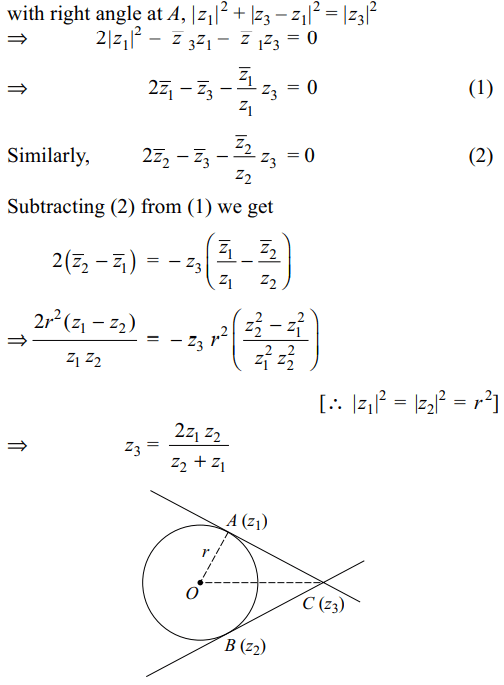8. Let $z=\cos\theta+i\sin\theta$    . Then value of $\sum_{m=1}^{15}Im\left(z^{2m-1}\right)$
at $\theta$ = 3° is
a) -1
b) 0
c) $\frac{1}{2\sin 3^{\circ}}$
d) $\frac{-1}{2\sin 3^{\circ}}$

Explanation:9. Let z = x + iy be a complex numbers where x and y are integers. Then area of the rectangle whose vertices are roots of $z\bar{z}^{3}+\bar{z}z^{3}=738$     is
a) 80
b) 48
c) 56
d) 96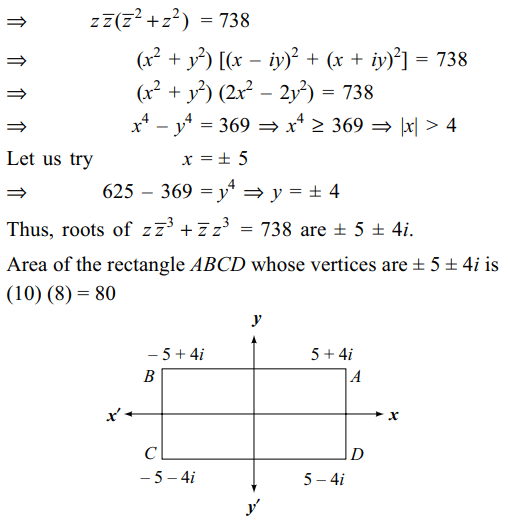a) $3e^{i\pi/4}+4i$
b) $\left(3-4\right)e^{i\pi/4}$
c) $\left(4+3i\right)e^{i\pi/4}$
d) $\left(3+4i\right)e^{i\pi/4}$